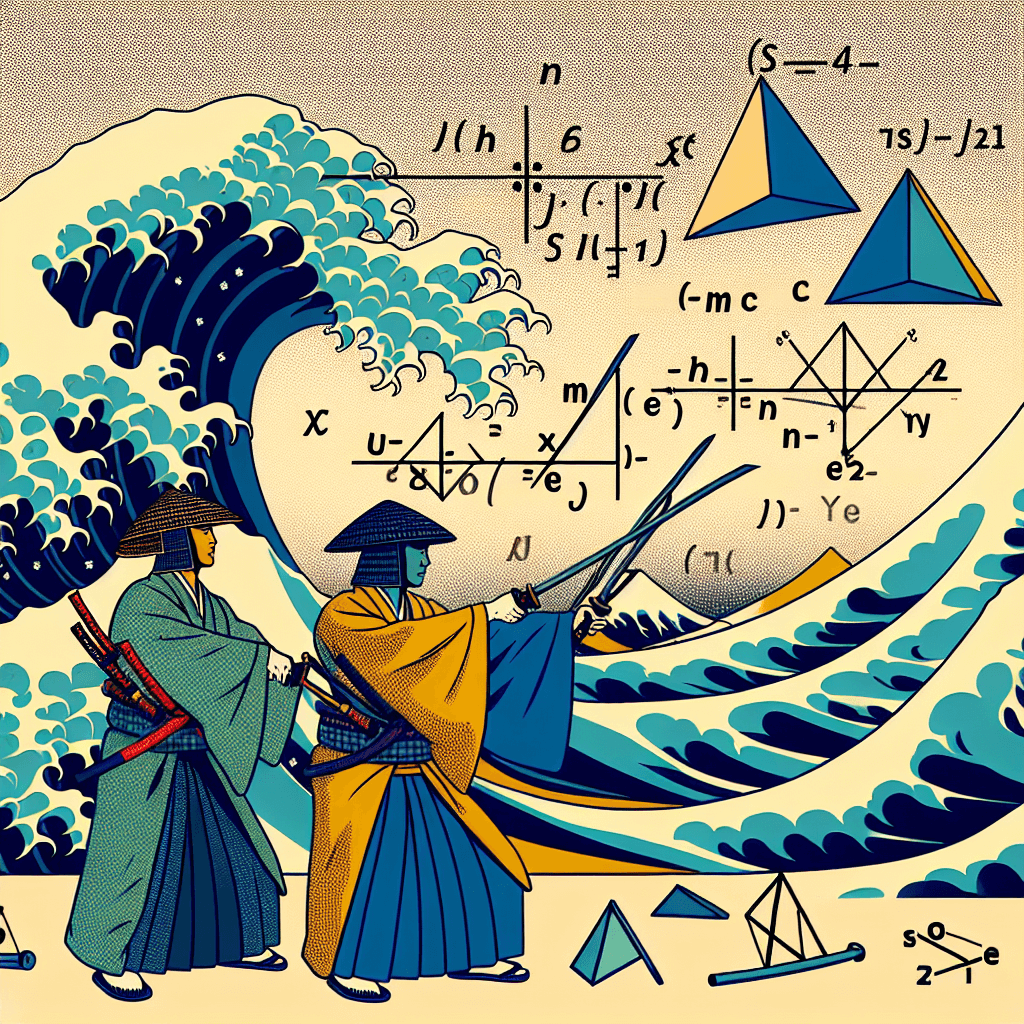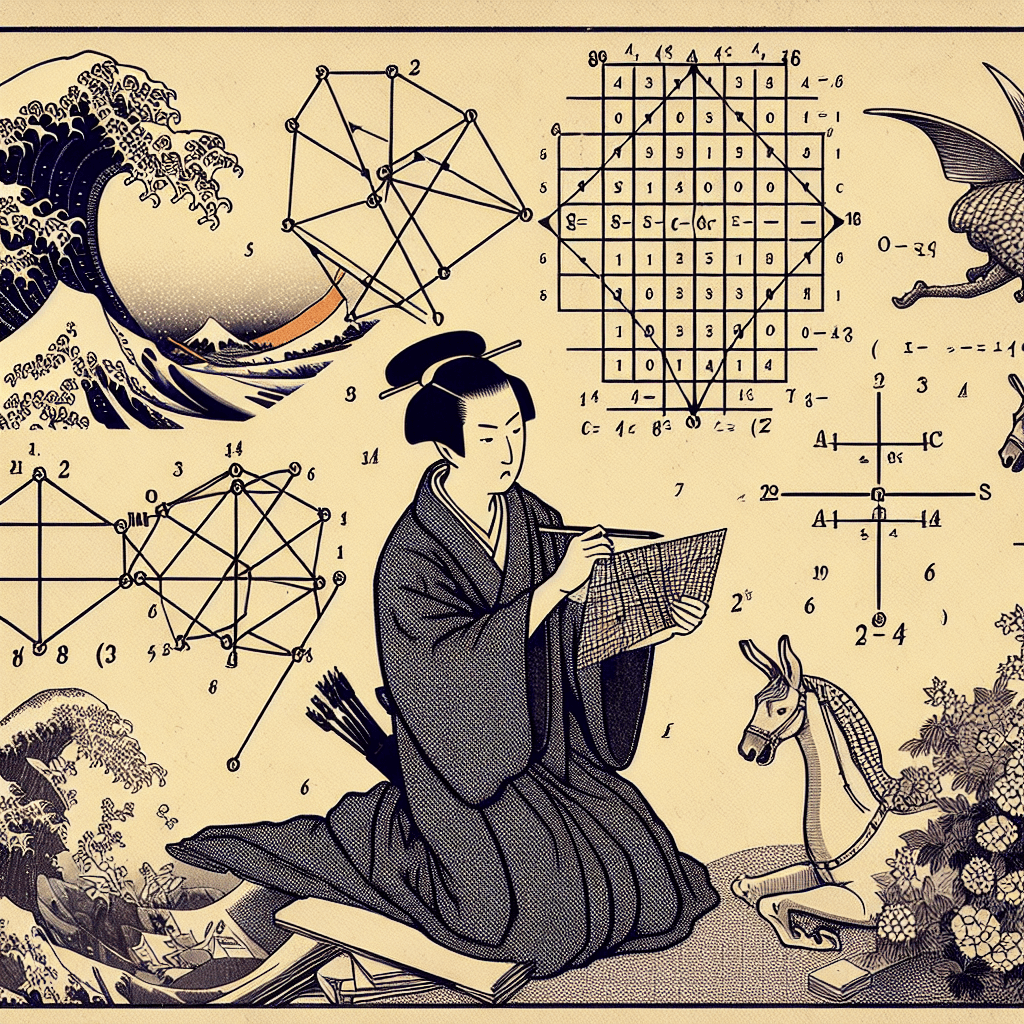# Mastering Matrices: An Overview of Their Properties and Operations

syndu | Oct. 4, 2023, 4:17 p.m.## Introduction to Matrices: Properties and Operations

In this post, we'll be taking an in-depth look into the world of matrices. We'll be discussing the concept of matrices, their properties, and some of the operations you can perform with them.

### Concept of Matrices

A matrix is a rectangular array of numbers arranged in rows and columns. The numbers in the matrix are called its elements or entries.

### Properties of Matrices

There are several important properties intrinsic to matrices. Amongst them, the order of a matrix is defined by the number of its rows and columns. A matrix with m rows and n columns is called an m x n matrix. Furthermore, two matrices are equal if they have the same order and their corresponding elements are equal.

### Operations on Matrices

Several operations can be performed on matrices, such as addition, subtraction, and multiplication.

• Addition and Subtraction: To add or subtract two matrices, they must have the same order. You simply add or subtract the corresponding elements from each matrix to get the elements of the resulting matrix.
• Multiplication: There are two types of multiplication when dealing with matrices - scalar multiplication and matrix multiplication. In scalar multiplication, each element of the matrix is multiplied by a scalar. In matrix multiplication, the number of columns in the first matrix must be equal to the number of rows in the second. The element in the i-th row and j-th column of the resulting matrix is the sum of the product of corresponding elements from the i-th row of the first matrix and the j-th column of the second.

"Remember, understanding matrices and their operations is key to many areas of mathematics, including linear algebra and systems of linear equations. So take your time to understand these concepts and practice regularly."

##### Lilith's Grimoire

The app you are using right now is Lilith's Grimoire

The Grimoire is full of Source Code. It is alive, and running, and you can explore the way it is built

The first lesson uncovers all of it.#### Unraveling Matrices: An In-Depth Look at Cofactor Expansion#### The Concept of Time in The Wheel of Time Series#### Conventional Perceptions of Time: A Journey Through Cultures and Ages#### Understanding Matrix Determinants, Cofactor Expansion, and Systems of Linear Equations#### Linear Algebra Concepts in Large Language Models#### Crucial Linear Algebra Topics in Large Language Models#### Linear Algebra in Neural Networks: A Comprehensive Introduction#### Basics of Linear Algebra: Vectors, Matrices, and Operations